gbdt_gbdt 算法 - CSDN

梯度提升决策树（Gradient Boosting Decision Tree，GBDT）算法是近年来被提及比较多的一个算法，这主要得益于其算法的性能，以及该算法在各类数据挖掘以及机器学习比赛中的卓越表现，有很多人对GBDT算法进行了开源代码的开发，比较火的是陈天奇的XGBoost和微软的LightGBM。

一、监督学习

1、监督学习的主要任务

监督学习是机器学习算法中重要的一种，对于监督学习，假设有m$m$个训练样本：

{(X(1),y(1)),(X(2),y(2)),⋯,(X(m),y(m))}\left \{ \left ( X^{\left ( 1 \right )},y^{\left ( 1 \right )} \right ),\left ( X^{\left ( 2 \right )},y^{\left ( 2 \right )} \right ),\cdots ,\left ( X^{\left ( m \right )},y^{\left ( m \right )} \right ) \right \}

其中，X(i)={x(i)1,x(i)2,⋯,x(i)m}$X^{\left ( i \right )}=\left \{ x^{\left ( i \right )}_1,x^{\left ( i \right )}_2,\cdots ,x^{\left ( i \right )}_m \right \}$称为第i$i$个样本的特征，y(1)$y^{\left ( 1 \right )}$称为第i$i$个样本的标签，样本标签可以为离散值，如分类问题；也可以为连续值，如回归问题。在监督学习中，利用训练样本训练出模型，该模型能够实现从样本特征X(i)$X^{\left ( i \right )}$到样本标签y(1)$y^{\left ( 1 \right )}$的映射，即：

X(i)→Fy(i)X^{\left ( i \right )}\overset{F}{\rightarrow} y^{\left ( i \right )}

为了能够对映射F(X)$F\left ( X \right )$进行求解，通常对模型设置损失函数L(y,F(X))$L\left ( y,F\left ( X \right ) \right )$，并求得在损失函数最小的情况下的映射为最好的映射：

F∗=argminF(X)L(y,F(X))F^\ast =\underset{F\left ( X \right )}{argmin}\; L\left ( y,F\left ( X \right ) \right )

对于一个具体的问题，如线性回归问题，其映射函数的形式为：

F(X;W)=WX=w0+w1x1+w2x2+⋯+wnxnF\left ( X;W \right )=WX=w_0+w_1x_1+w_2x_2+\cdots +w_nx_n

此时对于最优映射函数F(X;W)$F\left ( X;W \right )$的求解，实质是对映射函数中的参数W$W$的求解。对于参数的求解方法有很多，如梯度下降法。

2、梯度下降法

minf(w)min\; f\left ( w \right )

其基本步骤为：

随机选择一个初始点w0$w_0$
重复以下过程：
决定下降的方向：di=−∂∂wf(w)∣wi$d_i=-\frac{\partial }{\partial w}f\left ( w \right )\mid _{w_i}$
选择步长ρ$\rho$
更新：wi+1=wi+ρ⋅di$w_{i+1}=w_i+\rho \cdot d_i$
直到满足终止条件

梯度下降法的具体过程如下图所示：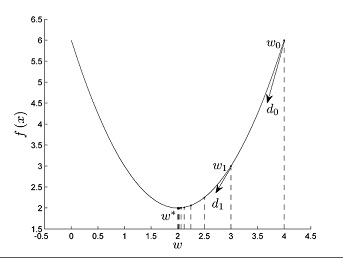由以上的过程，我们可以看出，对于最终的最优解w∗$w^\ast$，是由初始值w0$w_0$经过M$M$代的迭代之后得到的，在这里，设w0=d0$w_0=d_0$，则w∗$w^\ast$为：

w∗=∑i=0Mρi⋅diw^\ast =\sum_{i=0}^{M}\rho _i\cdot d_i

3、在函数空间的优化

以上是在指定的函数空间中对最优函数进行搜索，那么，能否直接在函数空间（function space）中查找到最优的函数呢？根据上述的梯度下降法的思路，对于模型的损失函数L(y,F(X))$L\left ( y,F\left ( X \right ) \right )$，为了能够求解出最优的函数F∗(X)$F^\ast\left ( X \right )$，首先，设置初始值为：

F0(X)=f0(X)F_0\left ( X \right )=f_0\left ( X \right )

以函数F(X)$F\left ( X \right )$作为一个整体，对于每一个样本X(i)$X^{\left ( i\right )}$，都存在对应的函数值F(X(i))$F\left ( X^{\left ( i\right )} \right )$。与梯度下降法的更新过程一致，假设经过M$M$代，得到最有的函数F∗(X)$F^\ast\left ( X \right )$为：

F∗(X)=∑i=0Mfi(X)F^\ast\left ( X \right )=\sum_{i=0}^{M}f_i\left ( X \right )

其中，fi(X)$f_i\left ( X \right )$为：

fi(X)=−ρigm(X)f_i\left ( X \right )=-\rho _ig_m\left ( X \right )

其中，gm(X)=[∂L(y,F(X))∂F(X)]F(X)=Fm−1(X)$g_m\left ( X \right )=\left [ \frac{\partial L\left ( y,F\left ( X \right ) \right )}{\partial F\left ( X \right )} \right ]_{F\left ( X \right )=F_{m-1}\left ( X \right )}$。

由上述的过程可以得到函数F(X)$F\left ( X \right )$的更新过程：

Fm(X)=∑i=0mfi(X)F_m\left ( X \right )=\sum_{i=0}^{m}f_i\left ( X \right )

与上面类似，函数f(X)$f\left ( X \right )$是由参数a$\textbf{a}$决定的，即：

f(X)=−ρ⋅h(X;a)f\left ( X \right )=-\rho \cdot h\left ( X;\textbf{a} \right )

二、Boosting

1、集成方法之Boosting

Boosting方法是集成学习中重要的一种方法，在集成学习方法中最主要的两种方法为Bagging和Boosting，在Bagging中，通过对训练样本重新采样的方法得到不同的训练样本集，在这些新的训练样本集上分别训练学习器，最终合并每一个学习器的结果，作为最终的学习结果，Bagging方法的具体过程如下图所示：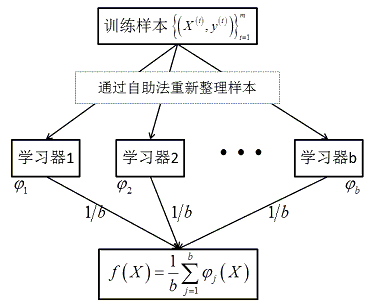在Bagging方法中，最重要的算法为随机森林Random Forest算法。由以上的图中可以看出，在Bagging方法中，b$b$个学习器之间彼此是相互独立的，这样的特点使得Bagging方法更容易并行。与Bagging方法不同，在Boosting算法中，学习器之间是存在先后顺序的，同时，每一个样本是有权重的，初始时，每一个样本的权重是相等的。首先，第1$1$个学习器对训练样本进行学习，当学习完成后，增大错误样本的权重，同时减小正确样本的权重，再利用第2$2$个学习器对其进行学习，依次进行下去，最终得到b$b$个学习器，最终，合并这b$b$个学习器的结果，同时，与Bagging中不同的是，每一个学习器的权重也是不一样的。Boosting方法的具体过程如下图所示：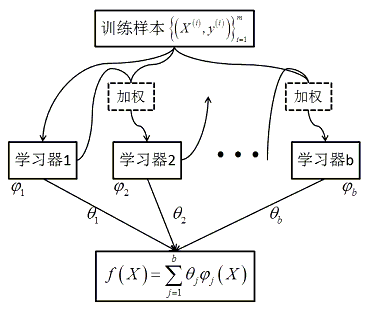由上图所示的Boosting方法中，最终的预测结果为b$b$个学习器结果的合并：

f(X)=∑j=1bθjφj(X)f\left ( X \right )=\sum_{j=1}^{b}\theta _j\varphi _j\left ( X \right )

这与上述的在函数空间中的优化类似：

Fm(X)=∑i=0m−ρi⋅h(X;ai)F_m\left ( X \right )=\sum_{i=0}^{m}-\rho _i\cdot h\left ( X;\textbf{a}_i  \right )

根据如上的函数空间中的优化可知，每次对每一个样本的训练的值为：

y¯i=⎡⎣⎢∂L(yi,F(X(i)))∂F(X(i))⎤⎦⎥F(X)=Fm−1(X)\bar{y}_i=\left [ \frac{\partial L\left ( y_i,F\left ( X^{\left ( i \right )} \right ) \right )}{\partial F\left ( X^{\left ( i \right )} \right )} \right ]_{F\left ( X \right )=F_{m-1}\left ( X \right )}

上建立模型，由于上述是一个求解梯度的过程，因此也称为基于梯度的Boost方法，其具体过程如下所示：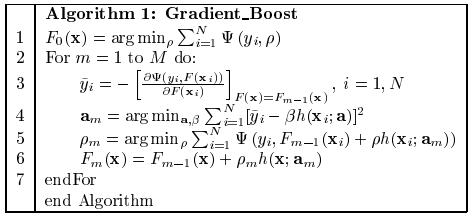1、分类回归树CART

分类回归树CART算法是一种基于二叉树的机器学习算法，其既能处理回归问题，又能处理分类为题，在梯度提升决策树GBDT算法中，使用到的是CART回归树算法，对于CART树算法的更多信息，可以参考简单易学的机器学习算法——分类回归树CART。

对于一个包含了m$m$个训练样本的回归问题，其训练样本为：

{(X(1),y(1)),(X(2),y(2)),⋯,(X(m),y(m))}\left \{ \left ( X^{\left ( 1 \right )},y^{\left ( 1 \right )} \right ),\left ( X^{\left ( 2 \right )},y^{\left ( 2 \right )} \right ),\cdots ,\left ( X^{\left ( m \right )},y^{\left ( m \right )} \right ) \right \}

其中，X(i)$X^{\left ( i \right )}$为n$n$维向量，表示的是第i$i$个样本的特征，y(i)$y^{\left ( i \right )}$为样本的标签，在回归问题中，标签y(i)$y^{\left ( i \right )}$为一系列连续的值。此时，利用训练样本训练一棵CART回归树：

开始时，CART树中只包含了根结点，所有样本都被划分在根结点上：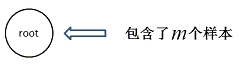此时，计算该节点上的样本的方差（此处要乘以m$m$），方差表示的是数据的波动程度。那么，根节点的方差的m$m$倍为：

s2⋅m=(y(1)−y¯)2+(y(2)−y¯)2+⋯+(y(m)−y¯)2s^2\cdot m=\left ( y^{\left ( 1 \right )}-\bar{y} \right )^2+\left ( y^{\left ( 2 \right )}-\bar{y} \right )^2+\cdots +\left ( y^{\left ( m \right )}-\bar{y} \right )^2

其中，y¯$\bar{y}$为标签的均值。此时，从n$n$维特征中选择第j$j$维特征，从m$m$个样本中选择一个样本的值：xj$x_j$作为划分的标准，当样本i$i$的第j$j$维特征小于等于xj$x_j$时，将样本划分到左子树中，否则，划分到右子树中，通过以上的操作，划分到左子树中的样本个数为m1$m_1$，划分到右子树的样本的个数为m2=m−m1$m_2=m-m_1$，其划分的结果如下图所示：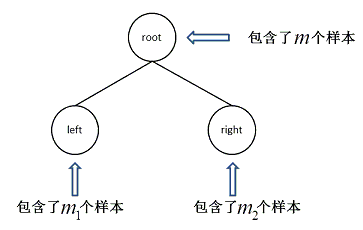那么，什么样本的划分才是当前的最好划分呢？此时计算左右子树的方差之和：s21⋅m1+s22⋅m2$s_1^2\cdot m_1+s_2^2\cdot m_2$：

s21⋅m1+s22⋅m2=∑X(i)∈left(y(i)−y¯1)2+∑X(j)∈right(y(j)−y¯2)2s_1^2\cdot m_1+s_2^2\cdot m_2=\sum_{X^{\left ( i \right )}\in left}\left ( y^{\left ( i \right )}-\bar{y}_1 \right )^2+\sum_{X^{\left ( j \right )}\in right}\left ( y^{\left ( j \right )}-\bar{y}_2 \right )^2

其中，y¯1$\bar{y}_1$为左子树中节点标签的均值，同理，y¯2$\bar{y}_2$为右子树中节点标签的均值。选择其中s21⋅m1+s22⋅m2$s_1^2\cdot m_1+s_2^2\cdot m_2$最小的划分作为最终的划分，依次这样划分下去，直到得到最终的划分，划分的结果为：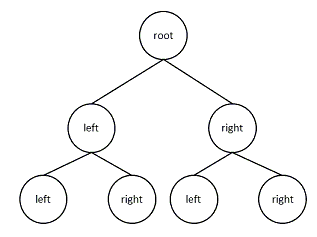注意：对于上述最优划分标准的选择，以上的计算过程可以进一步优化。

首先，对于s2⋅m$s^2\cdot m$：

s2⋅m=∑X(i)(y(i)−y¯)2=∑X(i)((y(i))2−2y(i)⋅y¯+(y¯)2)=∑X(i)(y(i))2−2m⎛⎝∑X(i)y(i)⎞⎠2+1m⎛⎝∑X(i)y(i)⎞⎠2=∑X(i)(y(i))2−1m⎛⎝∑X(i)y(i)⎞⎠2\begin{align*}
s^2\cdot m &=\sum_{X^{\left ( i \right )}}\left ( y^{\left ( i \right )}-\bar{y} \right )^2\\
&= \sum_{X^{\left ( i \right )}}\left ( \left ( y^{\left ( i \right )} \right )^2-2y^{\left ( i \right )}\cdot \bar{y}+\left ( \bar{y} \right )^2\right )\\
&=\sum_{X^{\left ( i \right )}}\left ( y^{\left ( i \right )} \right )^2-\frac{2}{m}\left ( \sum_{X^{\left ( i \right )}} y^{\left ( i \right )} \right )^2+\frac{1}{m}\left ( \sum_{X^{\left ( i \right )}} y^{\left ( i \right )} \right )^2 \\
&= \sum_{X^{\left ( i \right )}}\left ( y^{\left ( i \right )} \right )^2-\frac{1}{m}\left ( \sum_{X^{\left ( i \right )}} y^{\left ( i \right )} \right )^2
\end{align*}

而对于s21⋅m1+s22⋅m2$s_1^2\cdot m_1+s_2^2\cdot m_2$：

s21⋅m1+s22⋅m2=∑X(i)∈left(y(i)−y¯1)2+∑X(j)∈right(y(j)−y¯2)2=∑X(i)∈left((y(i))2−2y(i)⋅y¯1+(y¯1)2)+∑X(j)∈right((y(j))2−2y(j)⋅y¯2+(y¯2)2)\begin{align*}
s_1^2\cdot m_1+s_2^2\cdot m_2 &= \sum_{X^{\left ( i \right )}\in left}\left ( y^{\left ( i \right )}-\bar{y}_1 \right )^2+\sum_{X^{\left ( j \right )}\in right}\left ( y^{\left ( j \right )}-\bar{y}_2 \right )^2\\
&= \sum_{X^{\left ( i \right )}\in left}\left ( \left ( y^{\left ( i \right )} \right )^2-2y^{\left ( i \right )}\cdot \bar{y}_1+\left ( \bar{y}_1  \right )^2\right )+\sum_{X^{\left ( j \right )}\in right}\left ( \left ( y^{\left ( j \right )} \right )^2-2y^{\left ( j \right )}\cdot \bar{y}_2+\left ( \bar{y}_2  \right )^2 \right )
\end{align*}

=∑X(i)(y(i))2−2m1⎛⎝∑X(i)∈lefty(i)⎞⎠2+1m1⎛⎝∑X(i)∈lefty(i)⎞⎠2−2m2⎛⎝∑X(j)∈righty(j)⎞⎠2+1m2⎛⎝∑X(j)∈righty(j)⎞⎠2=∑X(i)(y(i))2−1m1⎛⎝∑X(i)∈lefty(i)⎞⎠2−1m2⎛⎝∑X(j)∈righty(j)⎞⎠2\begin{align*}
&=\sum_{X^{\left ( i \right )}}\left ( y^{\left ( i \right )} \right )^2-\frac{2}{m_1}\left ( \sum_{X^{\left ( i \right )}\in left} y^{\left ( i \right )} \right )^2+\frac{1}{m_1}\left ( \sum_{X^{\left ( i \right )}\in left} y^{\left ( i \right )} \right )^2 \\
&- \frac{2}{m_2}\left ( \sum_{X^{\left ( j \right )}\in right} y^{\left ( j \right )} \right )^2+\frac{1}{m_2}\left ( \sum_{X^{\left ( j \right )}\in right} y^{\left ( j \right )} \right )^2\\
&= \sum_{X^{\left ( i \right )}}\left ( y^{\left ( i \right )} \right )^2-\frac{1}{m_1}\left ( \sum_{X^{\left ( i \right )}\in left} y^{\left ( i \right )} \right )^2-\frac{1}{m_2}\left ( \sum_{X^{\left ( j \right )}\in right} y^{\left ( j \right )} \right )^2
\end{align*}

通过以上的过程，我们发现，划分前，记录节点的值为：

1m⎛⎝∑X(i)y(i)⎞⎠2\frac{1}{m}\left ( \sum_{X^{\left ( i \right )}} y^{\left ( i \right )} \right )^2

当划分后，两个节点的值的和为：

1m1⎛⎝∑X(i)∈lefty(i)⎞⎠2+1m2⎛⎝∑X(j)∈righty(j)⎞⎠2\frac{1}{m_1}\left ( \sum_{X^{\left ( i \right )}\in left} y^{\left ( i \right )} \right )^2+\frac{1}{m_2}\left ( \sum_{X^{\left ( j \right )}\in right} y^{\left ( j \right )} \right )^2

最好的划分，对应着两个节点的值的和的最大值。

2、GBDT——二分类

在梯度提升决策树GBDT中，通过定义不同的损失函数，可以完成不同的学习任务，二分类是机器学习中一类比较重要的分类算法，在二分类中，其损失函数为：

L(y,F)=log(1+exp(−2yF)),y∈{−1,1}L\left ( y,F \right )=log\left ( 1+exp\left ( -2yF \right ) \right ),\; y\in\left \{ -1,1 \right \}

套用上面介绍的GB框架，得到下述的二分类GBDT的算法：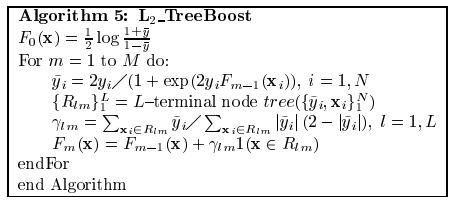在构建每一棵CART回归树的过程中，对一个样本的预测值应与y~$\tilde{y}$尽可能一致，对于y~$\tilde{y}$，其计算过程为：

y~(i)=−⎡⎣⎢∂L(y(i),F(X(i)))∂F(X(i))⎤⎦⎥F(X)=Fm−1(X)=−⎡⎣⎢∂log(1+exp(−2y(i)F(X(i))))∂F(X(i))⎤⎦⎥F(X)=Fm−1(X)=−⎡⎣⎢11+exp(−2y(i)F(X(i)))⋅exp(−2y(i)F(X(i)))⋅(−2y(i))⎤⎦⎥F(X)=Fm−1(X)\begin{align*}
\tilde{y}^{\left (i  \right )} &= -\left [ \frac{\partial L\left ( y^{\left ( i \right )},F\left ( X^{\left ( i \right )} \right ) \right )}{\partial F\left ( X^{\left ( i \right )} \right )} \right ]_{F\left ( X \right )=F_{m-1}\left ( X \right )}\\
&= -\left [ \frac{\partial log\left ( 1+exp\left ( -2y^{\left ( i \right )}F\left ( X^{\left ( i \right )} \right ) \right ) \right )}{\partial F\left ( X^{\left ( i \right )} \right )} \right ]_{F\left ( X \right )=F_{m-1}\left ( X \right )}\\
&= -\left [ \frac{1}{1+exp\left ( -2y^{\left ( i \right )}F\left ( X^{\left ( i \right )} \right ) \right )}\cdot exp\left ( -2y^{\left ( i \right )}F\left ( X^{\left ( i \right )} \right ) \right )\cdot \left ( -2y^{\left ( i \right )} \right ) \right ]_{F\left ( X \right )=F_{m-1}\left ( X \right )}
\end{align*}

=2y(i)⋅exp(−2y(i)F(X(i)))1+exp(−2y(i)F(X(i)))F(X)=Fm−1(X)=2y(i)1+exp(2y(i)Fm−1(X(i)))\begin{align*}
&=\frac{2y^{\left ( i \right )}\cdot exp\left ( -2y^{\left ( i \right )}F\left ( X^{\left ( i \right )} \right ) \right )}{1+exp\left ( -2y^{\left ( i \right )}F\left ( X^{\left ( i \right )} \right ) \right )}_{F\left ( X \right )=F_{m-1}\left ( X \right )}\\
&= \frac{2y^{\left ( i \right )}}{1+exp\left ( 2y^{\left ( i \right )}F_{m-1}\left ( X^{\left ( i \right )} \right ) \right )}
\end{align*}

在y~$\tilde{y}$（通常有的地方称为残差，在这里，更准确的讲是梯度下降的方向）上构建CART回归树。最终将每一个训练样本划分到对应的叶子节点中，计算此时该叶子节点的预测值：

γjm=argminγ∑X(i)∈Rjmlog(1+exp(−2y(i)(Fm−1(X(i))+γ)))\gamma _{jm}=\underset{\gamma }{argmin}\sum _{X^{\left ( i \right )}\in R_{jm}}log\left ( 1+exp\left ( -2y^{\left ( i \right )}\left ( F_{m-1}\left ( X^{\left ( i \right )} \right )+\gamma  \right ) \right ) \right )

由Newton-Raphson迭代公式可得：

γjm=∑X(i)∈Rjmy~(i)∑X(i)∈Rjm∣∣y~(i)∣∣(2−∣∣y~(i)∣∣)\gamma _{jm}=\frac{\sum _{X^{\left ( i \right )}\in R_{jm}}\tilde{y}^{\left (i  \right )}}{\sum _{X^{\left ( i \right )}\in R_{jm}}\left | \tilde{y}^{\left (i  \right )} \right |\left ( 2-\left | \tilde{y}^{\left (i  \right )} \right | \right )}

以参考文献3 Idiots’ Approach for Display Advertising Challenge中提供的代码为例：

GBDT训练的主要代码为：

void GBDT::fit(Problem const &Tr, Problem const &Va)
{
bias = calc_bias(Tr.Y); //用于初始化的F

std::vector<float> F_Tr(Tr.nr_instance, bias), F_Va(Va.nr_instance, bias);

Timer timer;
printf("iter     time    tr_loss    va_loss\n");
// 开始训练每一棵CART树
for(uint32_t t = 0; t < trees.size(); ++t)
{
timer.tic();

std::vector<float> const &Y = Tr.Y;
std::vector<float> R(Tr.nr_instance), F1(Tr.nr_instance); // 记录残差和F

#pragma omp parallel for schedule(static)
for(uint32_t i = 0; i < Tr.nr_instance; ++i)
R[i] = static_cast<float>(Y[i]/(1+exp(Y[i]*F_Tr[i]))); //计算残差，或者称为梯度下降的方向

// 利用上面的残差值，在此函数中构造一棵树
trees[t].fit(Tr, R, F1); // 分类树的生成

double Tr_loss = 0;
// 用上面训练的结果更新F_Tr，并计算log_loss
#pragma omp parallel for schedule(static) reduction(+: Tr_loss)
for(uint32_t i = 0; i < Tr.nr_instance; ++i)
{
F_Tr[i] += F1[i];
Tr_loss += log(1+exp(-Y[i]*F_Tr[i]));
}
Tr_loss /= static_cast<double>(Tr.nr_instance);

// 用上面训练的结果预测测试集，打印log_loss
#pragma omp parallel for schedule(static)
for(uint32_t i = 0; i < Va.nr_instance; ++i)
{
std::vector<float> x = construct_instance(Va, i);
F_Va[i] += trees[t].predict(x.data()).second;
}

double Va_loss = 0;
#pragma omp parallel for schedule(static) reduction(+: Va_loss)
for(uint32_t i = 0; i < Va.nr_instance; ++i)
Va_loss += log(1+exp(-Va.Y[i]*F_Va[i]));
Va_loss /= static_cast<double>(Va.nr_instance);

printf("%4d %8.1f %10.5f %10.5f\n", t, timer.toc(), Tr_loss, Va_loss);
fflush(stdout);
}
}

CART回归树的训练代码为：

void CART::fit(Problem const &prob, std::vector<float> const &R, std::vector<float> &F1){
uint32_t const nr_field = prob.nr_field; // 特征的个数
uint32_t const nr_sparse_field = prob.nr_sparse_field;
uint32_t const nr_instance = prob.nr_instance; // 样本的个数

std::vector<Location> locations(nr_instance); // 样本信息

#pragma omp parallel for schedule(static)
for(uint32_t i = 0; i < nr_instance; ++i)
locations[i].r = R[i]; // 记录每一个样本的残差

for(uint32_t d = 0, offset = 1; d < max_depth; ++d, offset *= 2){// d:深度

uint32_t const nr_leaf = static_cast<uint32_t>(pow(2, d)); // 叶子节点的个数

std::vector<Meta> metas0(nr_leaf); // 叶子节点的信息

for(uint32_t i = 0; i < nr_instance; ++i){

Location &location = locations[i]; //第i个样本的信息

if(location.shrinked)
continue;

Meta &meta = metas0[location.tnode_idx-offset]; //找到对应的叶子节点

meta.s += location.r; //残差之和
++meta.n;
}

std::vector<Defender> defenders(nr_leaf*nr_field); //记录每一个叶节点的每一维特征
std::vector<Defender> defenders_sparse(nr_leaf*nr_sparse_field);
// 针对每一个叶节点

for(uint32_t f = 0; f < nr_leaf; ++f){

Meta const &meta = metas0[f]; // 叶子节点

double const ese = meta.s*meta.s/static_cast<double>(meta.n); //该叶子节点的ese

for(uint32_t j = 0; j < nr_field; ++j)
defenders[f*nr_field+j].ese = ese;

for(uint32_t j = 0; j < nr_sparse_field; ++j)
defenders_sparse[f*nr_sparse_field+j].ese = ese;
}

std::vector<Defender> defenders_inv = defenders;

std::ref(metas0), std::ref(defenders), offset, true);
std::ref(metas0), std::ref(defenders_inv), offset, false);
scan_sparse(prob, locations, metas0, defenders_sparse, offset, true);

// 找出最佳的ese，scan里是每个字段的最佳ese，这里是所有字段的最佳ese，赋值给相应的tnode
for(uint32_t f = 0; f < nr_leaf; ++f){
// 对于每一个叶节点都找到最好的划分
Meta const &meta = metas0[f];
double best_ese = meta.s*meta.s/static_cast<double>(meta.n);

TreeNode &tnode = tnodes[f+offset];
for(uint32_t j = 0; j < nr_field; ++j){

Defender defender = defenders[f*nr_field+j];//每一个叶节点都对应着所有的特征

if(defender.ese > best_ese)
{
best_ese = defender.ese;
tnode.feature = j;
tnode.threshold = defender.threshold;
}

defender = defenders_inv[f*nr_field+j];
if(defender.ese > best_ese)
{
best_ese = defender.ese;
tnode.feature = j;
tnode.threshold = defender.threshold;
}
}
for(uint32_t j = 0; j < nr_sparse_field; ++j)
{
Defender defender = defenders_sparse[f*nr_sparse_field+j];
if(defender.ese > best_ese)
{
best_ese = defender.ese;
tnode.feature = nr_field + j;
tnode.threshold = defender.threshold;
}
}
}

// 把每个instance都分配给树里的一个叶节点下
#pragma omp parallel for schedule(static)
for(uint32_t i = 0; i < nr_instance; ++i){

Location &location = locations[i];
if(location.shrinked)
continue;

uint32_t &tnode_idx = location.tnode_idx;
TreeNode &tnode = tnodes[tnode_idx];
if(tnode.feature == -1){
location.shrinked = true;
}else if(static_cast<uint32_t>(tnode.feature) < nr_field){

if(prob.Z[tnode.feature][i].v < tnode.threshold)
tnode_idx = 2*tnode_idx;
else
tnode_idx = 2*tnode_idx+1;
}else{
uint32_t const target_feature = static_cast<uint32_t>(tnode.feature-nr_field);
bool is_one = false;
for(uint64_t p = prob.SJP[i]; p < prob.SJP[i+1]; ++p)
{
if(prob.SJ[p] == target_feature)
{
is_one = true;
break;
}
}
if(!is_one)
tnode_idx = 2*tnode_idx;
else
tnode_idx = 2*tnode_idx+1;
}
}
}

// 用于计算gamma
std::vector<std::pair<double, double>>
tmp(max_tnodes, std::make_pair(0, 0));
for(uint32_t i = 0; i < nr_instance; ++i)
{
float const r = locations[i].r;
uint32_t const tnode_idx = locations[i].tnode_idx;
tmp[tnode_idx].first += r;
tmp[tnode_idx].second += fabs(r)*(1-fabs(r));
}

for(uint32_t tnode_idx = 1; tnode_idx <= max_tnodes; ++tnode_idx)
{
double a, b;
std::tie(a, b) = tmp[tnode_idx];
tnodes[tnode_idx].gamma = (b <= 1e-12)? 0 : static_cast<float>(a/b);
}

#pragma omp parallel for schedule(static)
for(uint32_t i = 0; i < nr_instance; ++i)
F1[i] = tnodes[locations[i].tnode_idx].gamma;// 重新更新F1的值
}

在参考文献A simple GBDT in Python中提供了Python实现的GBDT的版本。

参考文献

Greedy Function Approximation: A Gradient Boosting Machine
3 Idiots’ Approach for Display Advertising Challenge
《统计机器学习》
GBDT：梯度提升决策树
随机森林&GBDT算法以及在MLlib中的实现
A simple GBDT in Python

展开全文GBDT 机器学习
• ## GBDT算法原理以及实例理解

万次阅读 多人点赞 2020-07-17 14:04:40
【尊重原创，转载请注明出处】http://blog.csdn.net/zpalyq110/article/details/79527653

写在前面： 去年学习GBDT之初，为了加强对算法的理解，整理了一篇笔记形式的文章，发出去之后发现阅读量越来越多，渐渐也有了评论，评论中大多指出来了笔者理解或者编辑的错误，故重新编辑一版文章，内容更加翔实，并且在GitHub上实现了和本文一致的GBDT简易版（包括回归、二分类、多分类以及可视化），供大家交流探讨。感谢各位的点赞和评论，希望继续指出错误。
Github：https://github.com/Freemanzxp/GBDT_Simple_Tutorial

简介：
1. Decision Tree：CART回归树
首先，GBDT使用的决策树是CART回归树，无论是处理回归问题还是二分类以及多分类，GBDT使用的决策树通通都是都是CART回归树。为什么不用CART分类树呢？因为GBDT每次迭代要拟合的是梯度值，是连续值所以要用回归树。
对于回归树算法来说最重要的是寻找最佳的划分点，那么回归树中的可划分点包含了所有特征的所有可取的值。在分类树中最佳划分点的判别标准是熵或者基尼系数，都是用纯度来衡量的，但是在回归树中的样本标签是连续数值，所以再使用熵之类的指标不再合适，取而代之的是平方误差，它能很好的评判拟合程度。

回归树生成算法：
输入：训练数据集$D$:
输出：回归树$f(x)$.
在训练数据集所在的输入空间中，递归的将每个区域划分为两个子区域并决定每个子区域上的输出值，构建二叉决策树：
（1）选择最优切分变量$j$与切分点$s$，求解
$\underset{j,s}{min}\left [ \underset{c_1}{min}\underset{x_i\in R_1(j,s)}{\sum}(y_i-c_1)^2 + \underset{c_2}{min}\underset{x_i\in R_2(j,s)}{\sum}(y_i-c_2)^2\ \right ]$遍历变量$j$，对固定的切分变量$j$扫描切分点$s$，选择使得上式达到最小值的对$(j,s)$.
（2）用选定的对$(j,s)$划分区域并决定相应的输出值：$R_1(j,s)={x|x^{(j)}\leq s}, R_2(j,s)={x|x^{(j)}> s}$$\hat{c_m} =\frac{1}{N}\underset{x_1\in R_m(j,s)}{\sum}y_i, x \in R_m, m=1,2$（3）继续对两个子区域调用步骤（1）和（2），直至满足停止条件。
（4）将输入空间划分为$M$个区域 $R_1,R_2,...R_M$，生成决策树：
$f(x)=\sum_{m=1}^{M}\hat{c}_m I(x \in R_m)$

梯度提升树（Grandient Boosting）是提升树（Boosting Tree）的一种改进算法，所以在讲梯度提升树之前先来说一下提升树。

先来个通俗理解：假如有个人30岁，我们首先用20岁去拟合，发现损失有10岁，这时我们用6岁去拟合剩下的损失，发现差距还有4岁，第三轮我们用3岁拟合剩下的差距，差距就只有一岁了。如果我们的迭代轮数还没有完，可以继续迭代下面，每一轮迭代，拟合的岁数误差都会减小。最后将每次拟合的岁数加起来便是模型输出的结果。

提升树算法：
（1）初始化$f_0(x)=0$
（2）对$m=1,2,...,M$
（a）计算残差$r_{mi}=y_i-f_{m-1}(x), i=1,2,...,N$ （b）拟合残差$r_{mi}$学习一个回归树，得到$h_m(x)$
（c）更新$f_m(x) = f_{m-1}+h_m(x)$
（3）得到回归问题提升树$f_M(x)=\sum_{m=1}^Mh_m(x)$

上面伪代码中的残差是什么？
在提升树算法中，假设我们前一轮迭代得到的强学习器是$f_{t−1}(x)$损失函数是$L(y,f_{t−1}(x))$我们本轮迭代的目标是找到一个弱学习器$h_t(x)$最小化让本轮的损失$L(y,f_t(x))=L(y,f_{t−1}(x)+h_t(x))$当采用平方损失函数时$L(y,f_{t−1}(x)+h_t(x))$$=(y-f_{t−1}(x)-h_t(x))^2$$=(r-h_t(x))^2$这里，$r=y-f_{t−1}(x)$是当前模型拟合数据的残差（residual）所以，对于提升树来说只需要简单地拟合当前模型的残差。
回到我们上面讲的那个通俗易懂的例子中，第一次迭代的残差是10岁，第二 次残差4岁……

当损失函数是平方损失和指数损失函数时，梯度提升树每一步优化是很简单的，但是对于一般损失函数而言，往往每一步优化起来不那么容易，针对这一问题，Friedman提出了梯度提升树算法，这是利用最速下降的近似方法，其关键是利用损失函数的负梯度作为提升树算法中的残差的近似值。
那么负梯度长什么样呢？
第t轮的第i个样本的损失函数的负梯度为：$-\bigg[\frac{\partial L(y, f(x_i)))}{\partial f(x_i)}\bigg]_{f(x) = f_{t-1}(x)\;\;}$此时不同的损失函数将会得到不同的负梯度，如果选择平方损失$L(y,f(x_i)) = \frac{1}{2}(y-f(x_i))^2$负梯度为$-\bigg[\frac{\partial L(y, f(x_i)))}{\partial f(x_i)}\bigg]_{f(x) = f_{t-1}(x)\;\;}=y-f(x_i)$  此时我们发现GBDT的负梯度就是残差，所以说对于回归问题，我们要拟合的就是残差。
那么对于分类问题呢？二分类和多分类的损失函数都是$log loss$，本文以回归问题为例进行讲解。

3. GBDT算法原理

GBDT算法：
（1）初始化弱学习器
$f_0(x) = {arg\;min}_{c}\sum\limits_{i=1}^{N}L(y_i, c)$（2）对$m=1,2,...,M$有：
（a）对每个样本$i=1,2,...,N$，计算负梯度，即残差
$r_{im} = -\bigg[\frac{\partial L(y_i, f(x_i)))}{\partial f(x_i)}\bigg]_{f(x) = f_{m-1} (x)}$
（b）将上步得到的残差作为样本新的真实值，并将数据$(x_i,r_{im})， i=1,2,..N$作为下棵树的训练数据，得到一颗新的回归树$f_{m} (x)$其对应的叶子节点区域为$R_{jm}, j =1,2,..., J$。其中$J$为回归树t的叶子节点的个数。
（c）对叶子区域$j =1,2,..J$计算最佳拟合值
$\Upsilon_{jm} = \underbrace{arg\; min}_{\Upsilon}\sum\limits_{x_i \in R_{jm}} L(y_i,f_{m-1}(x_i) +\Upsilon)$ （d）更新强学习器
$f_{m}(x) = f_{m-1}(x) + \sum\limits_{j=1}^{J}\Upsilon_{jm}I(x \in R_{jm})$（3）得到最终学习器
$f(x) = f_M(x) =f_0(x) + \sum\limits_{m=1}^{M}\sum\limits_{j=1}^{J}\Upsilon_{jm}I(x \in R_{jm})$

4. 实例详解
本人用python以及pandas库实现GBDT的简易版本，在下面的例子中用到的数据都在github可以找到，大家可以结合代码和下面的例子进行理解，欢迎star~
Github：https://github.com/Freemanzxp/GBDT_Simple_Tutorial

数据介绍：
如下表所示：一组数据，特征为年龄、体重，身高为标签值。共有5条数据，前四条为训练样本，最后一条为要预测的样本。

编号
年龄(岁)
体重（kg）
身高(m)(标签值)

0
5
20
1.1

1
7
30
1.3

2
21
70
1.7

3
30
60
1.8

4(要预测的)
25
65
？

训练阶段：

参数设置：

学习率：learning_rate=0.1
迭代次数：n_trees=5
树的深度：max_depth=3

1.初始化弱学习器:$f_0(x) = {arg\;min}_{c}\sum\limits_{i=1}^{N}L(y_i, c)$  损失函数为平方损失，因为平方损失函数是一个凸函数，直接求导，倒数等于零，得到$c$。
$\sum\limits_{i=1}^{N}\frac{\partial L(y_i,c))}{\partial c}=\sum\limits_{i=1}^{N}\frac{\partial (\frac{1}{2}(y_i-c)^2)}{\partial c}=\sum\limits_{i=1}^{N}c-y_i$令导数等于0
$\sum\limits_{i=1}^{N}c-y_i=0$$c=(\sum\limits_{i=1}^{N}y_i)/N$所以初始化时，$c$取值为所有训练样本标签值的均值。$c=(1.1+1.3+1.7+1.8)/4=1.475$，此时得到初始学习器$f_0(x)$
$f_0(x) = c=1.475$

2.对迭代轮数m=1，2,…,M:
由于我们设置了迭代次数：n_trees=5，这里的$M=5$。
计算负梯度，根据上文损失函数为平方损失时，负梯度就是残差残差，再直白一点就是 $y$与上一轮得到的学习器$f_{m-1}$的差值
$r_{i1} = -\bigg[\frac{\partial L(y_i, f(x_i)))}{\partial f(x_i)}\bigg]_{f(x) = f_{0} (x)}$
残差在下表列出：

编号
真实值
$f_{0} (x)$
残差

0
1.1
1.475
-0.375

1
1.3
1.475
-0.175

2
1.7
1.475
0.225

3
1.8
1.475
0.325

此时将残差作为样本的真实值来训练弱学习器$f_{1} (x)$，即下表数据

编号
年龄(岁)
体重（kg）
标签值

0
5
20
-0.375

1
7
30
-0.175

2
21
70
0.225

3
30
60
0.325

接着，寻找回归树的最佳划分节点，遍历每个特征的每个可能取值。从年龄特征的5开始，到体重特征的70结束，分别计算分裂后两组数据的平方损失（Square Error），$SE_l$左节点平方损失，$SE_r$右节点平方损失，找到使平方损失和$SE_{sum}=SE_l+SE_r$最小的那个划分节点，即为最佳划分节点。
例如：以年龄7为划分节点，将小于7的样本划分为到左节点，大于等于7的样本划分为右节点。左节点包括$x_0$，右节点包括样本$x_1,x_2,x_3$，$SE_l = 0,SE_r=0.140,SE_{sum}=0.140$，所有可能划分情况如下表所示：

划分点
小于划分点的样本
大于等于划分点的样本
$SE_l$
$SE_r$
$SE_{sum}$

年龄5
/
0，1，2，3
0
0.327
0.327

年龄7
0
1，2，3
0
0.140
0.140

年龄21
0，1
2，3
0.020
0.005
0.025

年龄30
0，1，2
3
0.187
0
0.187

体重20
/
0，1，2，3
0
0.327
0.327

体重30
0
1，2，3
0
0.140
0.140

体重60
0，1
2，3
0.020
0.005
0.025

体重70
0，1，3
2
0.260
0
0.260

以上划分点是的总平方损失最小为0.025有两个划分点：年龄21和体重60，所以随机选一个作为划分点，这里我们选 年龄21
现在我们的第一棵树长这个样子：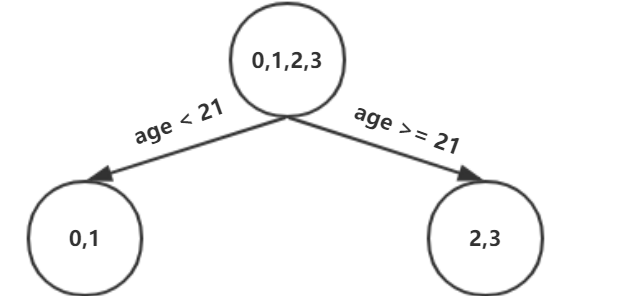我们设置的参数中树的深度max_depth=3，现在树的深度只有2，需要再进行一次划分，这次划分要对左右两个节点分别进行划分：

对于左节点，只含有0,1两个样本，根据下表我们选择年龄7划分

划分点
小于划分点的样本
大于等于划分点的样本
$SE_l$
$SE_r$
$SE_{sum}$

年龄5
/
0，1
0
0.020
0.020

年龄7
0
1
0
0
0

体重20
/
0，1
0
0.020
0.020

体重30
0
1
0
0
0

对于右节点，只含有2,3两个样本，根据下表我们选择年龄30划分（也可以选体重70）

划分点
小于划分点的样本
大于等于划分点的样本
$SE_l$
$SE_r$
$SE_{sum}$

年龄21
/
2，3
0
0.005
0.005

年龄30
2
3
0
0
0

体重60
/
2，3
0
0.005
0.005

体重70
3
2
0
0
0

现在我们的第一棵树长这个样子：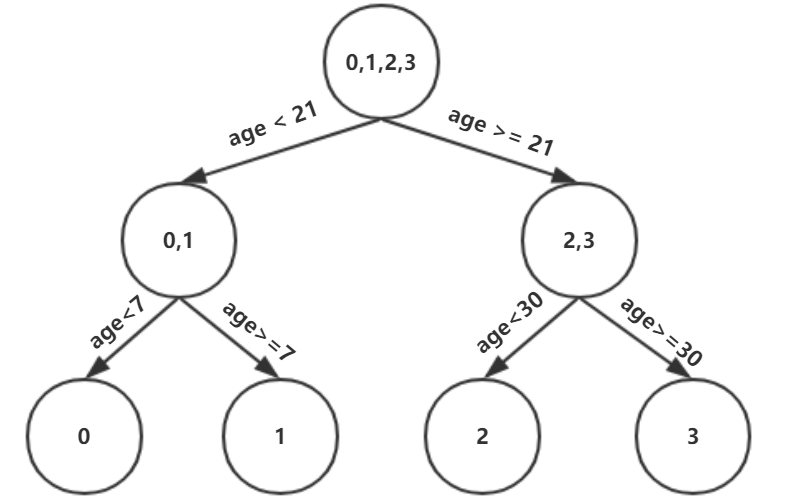此时我们的树深度满足了设置，还需要做一件事情，给这每个叶子节点分别赋一个参数$\Upsilon$，来拟合残差。$\Upsilon_{j1} = \underbrace{arg\; min}_{\Upsilon}\sum\limits_{x_i \in R_{j1}} L(y_i,f_{0}(x_i) +\Upsilon)$  这里其实和上面初始化学习器是一个道理，平方损失，求导，令导数等于零，化简之后得到每个叶子节点的参数$\Upsilon$，其实就是标签值的均值。这个地方的标签值不是原始的 $y$，而是本轮要拟合的标残差 $y-f_0(x)$.
根据上述划分结果，为了方便表示，规定从左到右为第$1,2,3,4$个叶子结点$(x_0 \in R_{11})，\Upsilon_{11}=-0.375$$(x_1 \in R_{21})，\Upsilon_{21}=-0.175$$(x_2 \in R_{31})，\Upsilon_{31}=0.225$$(x_3 \in R_{41})，\Upsilon_{41}=0.325$
此时的树长这个样子：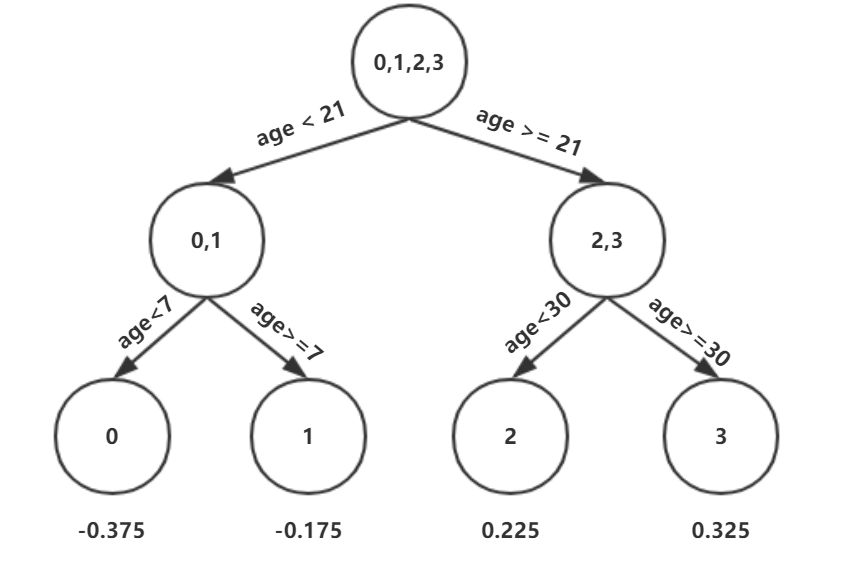此时可更新强学习器，需要用到参数学习率：learning_rate=0.1，用$lr$表示。
$f_{1}(x) = f_{0}(x) + lr *\sum\limits_{j=1}^{4}\Upsilon_{j1}I(x \in R_{j1})$
为什么要用学习率呢？这是Shrinkage的思想，如果每次都全部加上（学习率为1）很容易一步学到位导致过拟合。

重复此步骤，直到 $m>5$ 结束，最后生成5棵树。
下面将展示每棵树最终的结构，这些图都是GitHub上的代码生成的，感兴趣的同学可以去一探究竟
Github：https://github.com/Freemanzxp/GBDT_Simple_Tutorial
第一棵树：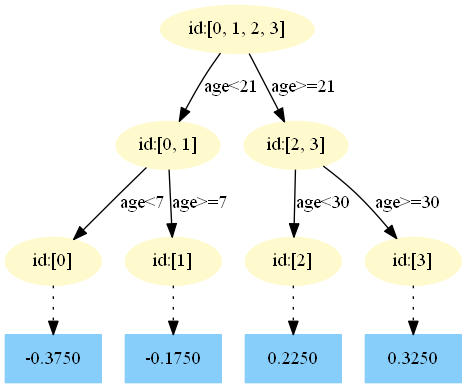第二棵树：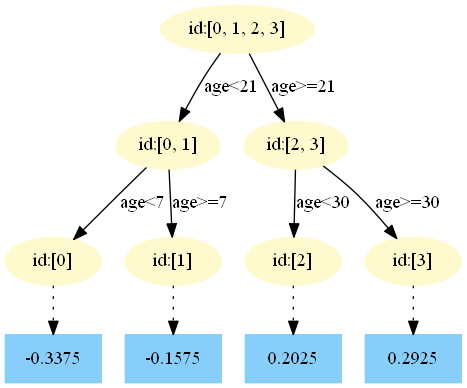第三棵树：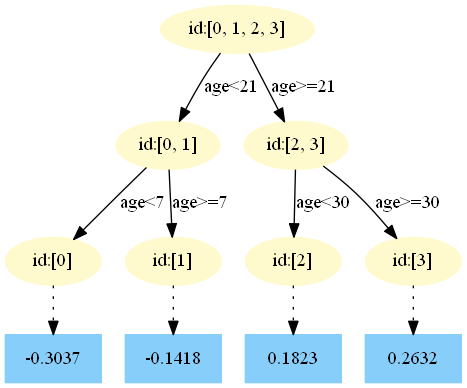第四棵树：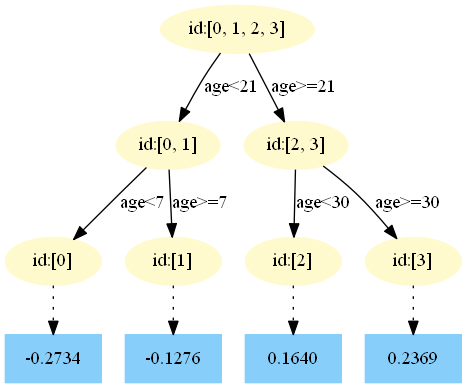第五棵树：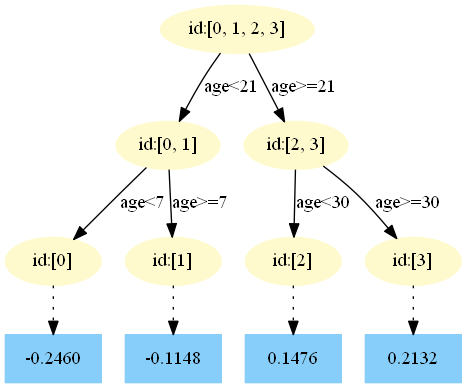4.得到最后的强学习器：
$f(x) = f_{5}(x) =f_0(x) + \sum\limits_{m=1}^{5}\sum\limits_{j=1}^{4}\Upsilon_{jm}I(x \in R_{jm})$

5.预测样本5：
$f_0(x)=1.475$
在$f_1(x)$中，样本4的年龄为25，大于划分节点21岁，又小于30岁，所以被预测为0.2250。
在$f_2(x)$中，样本4的…此处省略…所以被预测为0.2025
为什么是0.2025？这是根据第二颗树得到的，可以GitHub简单运行一下代码
在$f_3(x)$中，样本4的…此处省略…所以被预测为0.1823
在$f_4(x)$中，样本4的…此处省略…所以被预测为0.1640
在$f_5(x)$中，样本4的…此处省略…所以被预测为0.1476
最终预测结果：$f(x) =1.475+ 0.1 * (0.225+0.2025+0.1823+0.164+0.1476) = 1.56714$

4. 总结
本文章从GBDT算法的原理到实例详解进行了详细描述，但是目前只写了回归问题，GitHub上的代码也是实现了回归、二分类、多分类以及树的可视化，希望大家继续批评指正，感谢各位的关注。

参考资料

李航 《统计学习方法》
Friedman J H . Greedy Function Approximation: A Gradient Boosting Machine[J]. The Annals of Statistics, 2001, 29(5):1189-1232.

【尊重原创，转载请注明出处】 http://blog.csdn.net/zpalyq110/article/details/79527653


展开全文GBDT 梯度提升树 决策树 算法
• ## GBDT基本原理及算法描述

万次阅读 多人点赞 2018-08-26 13:24:24
一. 前言

当GBDT的损失函数是平方损失时，即时，则负梯度，而即为我们所说的残差，而我们的GBDT的思想就是在每次迭代中拟合残差来学习一个弱学习器。而残差的方向即为我们全局最优的方向。但是当损失函数不为平方损失时，我们该如何拟合弱学习器呢？大牛Friedman提出使用损失函数负梯度的方向代替残差方向，我们称损失函数负梯度为伪残差。而伪残差的方向即为我们局部最优的方向。所以在GBDT中，当损失函数不为平方损失时，用每次迭代的局部最优方向代替全局最优方向（这种方法是不是很熟悉？）。

说了这么多，现在举个例子来看看GBDT是如何拟合残差来学习弱学习器的。我们可以证明，当损失函数为平方损失时，叶节点中使平方损失误差达到最小值的是叶节点中所有值的均值；而当损失函数为绝对值损失时，叶节点中使绝对损失误差达到最小值的是叶节点中所有值的中位数。相关证明将在最后的附录中给出。

训练集是4个人，A，B，C，D年龄分别是14，16，24，26。样本中有购物金额、上网时长、经常到百度知道提问等特征。提升树的过程如下：（图片来源）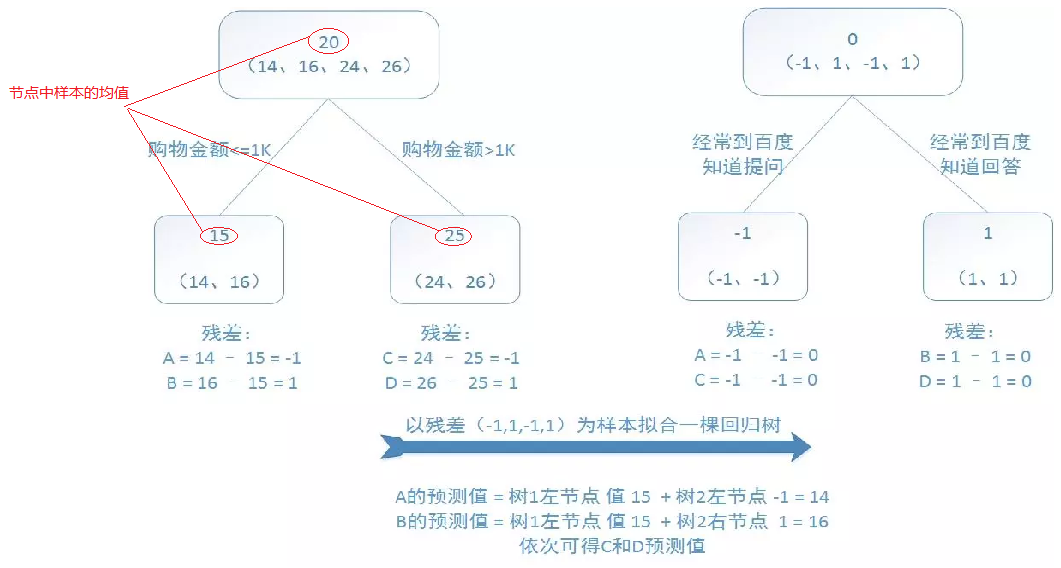从上图可以看出，第一棵树建立的时候使用的是原始数据，而后每一棵树建立使用的是前n-1次的残差来拟合弱学习器。

下面，我们就来简单的介绍一下GBDT的基本原理和算法描述。

二. GBDT回归树基本模版

梯度提升算法的回归树基本模版，如下所示：

输入：训练数据集，损失函数为

输出：回归树

（1）初始化：（估计使损失函数极小化的常数值，它是只有一个根节点的树，一般平方损失函数为节点的均值，而绝对损失函数为节点样本的中位数）

（2）对（M表示迭代次数，即生成的弱学习器个数）：

（a）对样本，计算损失函数的负梯度在当前模型的值将它作为残差的估计，对于平方损失函数为，它就是通常所说的残差；而对于一般损失函数，它就是残差的近似值（伪残差）：

（b）对拟合一个回归树，得到第m棵树的叶节点区域，（J表示每棵树的叶节点个数）

（c）对，利用线性搜索，估计叶节点区域的值，使损失函数最小化，计算

（d）更新

（3）得到最终的回归树

三. GBDT的算法描述

3.1 GBDT的损失函数

3.1.1 梯度提升回归树损失函数介绍

（1）ls：平方损失，这是最常见的回归损失函数了，如下：

对应负梯度为：

（3）huber：huber损失，它是平方损失和绝对损失的这种产物，对于远离中心的异常点采用绝对损失，而中心附近的点采用平方损失。这个界限一般用分位数点度量。损失函数如下：

对应的负梯度为：

（4）quantile：分位数损失，它对应的是分位数回归的损失函数，表达式如下：

其中θ为分位数，需要我们在回归前指定。对应的负梯度为：

对于huber损失和分位数损失主要作用就是减少异常点对损失函数的影响。

3.1.2 梯度提升分类树损失函数介绍

（1）exponential：指数损失，表达式如下：

（2）deviance：对数损失，类似于logistic回归的损失函数，输出的是类别的概率，表达式如下：

下面我们来分别的介绍一下，这几种损失函数对应GBDT算法。

3.2 GBDT回归算法描述

3.2.1 平方损失GBDT算法描述

输入：训练数据集，损失函数为

输出：回归树

（1）初始化：（可以证明当损失函数为平方损失时，节点的平均值即为该节点中使损失函数达到最小值的最优预测值,证明在最下面的附录给出）

（2）对：

（a）对样本，计算伪残差（对于平方损失来说，伪残差就是真残差）

，

（b）对拟合一个回归树，得到第m棵树的叶节点区域，

（c）对，利用线性搜索，估计叶节点区域的值，使损失函数最小化，计算

，K表示第m棵树的第j个节点中的样本数量

上式表示的取值为第m棵树的第j个叶节点中伪残差的平均数

（d）更新

（3）得到最终的回归树

3.2.2 绝对损失GBDT算法描述

输入：训练数据集，损失函数为

输出：回归树

（1）初始化：（可以证明当损失函数为绝对损失时，节点中样本的中位数即为该节点中使损失函数达到最小值的最优预测值,证明在最下面的附录给出）

（2）对：

（a）对样本，计算伪残差

，

（b）对拟合一个回归树，得到第m棵树的叶节点区域，

（c）对，，计算

上式表示的取值为第m棵树的第j个叶节点中伪残差的中位数

（d）更新

（3）得到最终的回归树

3.2.3 huber损失GBDT算法描述

输入：训练数据集，损失函数为

输出：回归树

（1）初始化：

（2）对：

（a）对样本，计算

表示分位数；表示将伪残差的百分之多少设为分位数，在sklearn中是需要我们自己设置的，默认为0.9

（b）对拟合一个回归树，得到第m棵树的叶节点区域，

（c）对，，计算

（d）更新

（3）得到最终的回归树

3.3 GBDT分类算法描述

3.3.1 log损失GBDT的二分类算法描述

输入：训练数据集，损失函数为，y={-1，1}

输出：分类树

（1）初始化：

（2）对：

（a）对样本，计算伪残差

（b）对概率残差拟合一个分类树，得到第m棵树的叶节点区域，

（c）对，，计算

（d）更新

（3）得到最终的分类树

由于我们用的是类别的预测概率值和真实概率值的差来拟合损失，所以最后还要讲概率转换为类别，如下：

最终输出比较类别概率大小，概率大的就预测为该类别。

3.3.2 log损失GBDT的多分类算法描述

输入：训练数据集，损失函数为，=｛0，1｝表示是否属于第k类别，1表示是，0表示否。，表示共有多少分类的类别。

输出：分类树

（1）初始化：

，

（2）对：

（a）计算样本点俗属于每个类别的概率：

（b）对k=1,2,...,K：

1） ，

2）对概率伪残差拟合一个分类树

3）

4）

（3）得到最终的分类树

最后得到的可以被用来去得到分为第k类的相应的概率：

由于我们用的是类别的预测概率值和真实概率值的差来拟合损失，所以最后还要将概率转换为类别，如下：

为最终的输出类别，为当真实值为时，预测为第k类时的联合代价，即概率最大的类别即为我们所预测的类别。当K=2时，该算法等价于为二分类算法。

到这里，我们算法的描述环节已经介绍完毕。还有一个算法就是分位数回归的算法描述没有介绍，因为早期的论文里面并没有介绍到该算法，所以，这里我们也不予以介绍，感兴趣的小伙伴可以查阅相关资料或者直接看sklearn有关该算法的源码。

最后，我们还有两个证明没有说，接下来我们证明我们在上面提到的有关损失函数为平方损失时叶节点的最佳预测为叶节点的残差均值和损失函数为绝对损失时，叶节点的最佳预测为叶节点残差的中位数。

四. 附录

4.1 证明：损失函数为平方损失时，叶节点的最佳预测为叶节点残差均值

节点R中有N个样本点，假设s为切分点，，分别为切分后的左节点和右节点，分别有节点个数为。

我们的目标是找到切分点s，在，内部使平方损失误差达到最小值的，如下：

和分别对求偏导，并令偏导等于0，得到在，内部使平方损失误差达到最小值的：

，

而和即为各自叶节点中的残差的均值。

4.2 证明：损失函数为绝对损失时，叶节点的最佳预测为叶节点残差的中位数。

损失函数

假设在节点中有个节点使，则有个节点使，那么：

我们的目标是是损失函数最小化，所以，上式对求偏导，并令偏导等于0，得：

得：

而N为节点中样本的总数，所以使节点的最佳预测为节点中残差的中位数。

五. 参考文献/博文

（1）大牛Friedman关于梯度提升的论文

（2）《统计学习方法》第八章

（3）关于机器学习总结比较好的博客


展开全文GBDT 梯度提升树
• 第二个模块通过GBDT的三要素：GB(梯度提升)，DT(回归树)和Shrinkage(缩减)理解GBDT的算法核心。 第三个模块通过剖析分类和回归损失函数来讲解GBDT在分类和回归方面的应用。 第四个模块通过手动方式一步步拆解...机器学习 机器学习算法 机器学习实战 python机器学习
• ## GBDT详解

千次阅读 2018-08-19 19:17:02
参考以下两篇博文：

http://blog.csdn.net/w28971023/article/details/8240756

https://www.cnblogs.com/ModifyRong/p/7744987.html

GBDT算法内部究竟是如何工作的？

gbdt 的算法的流程？
gbdt 如何选择特征 ？
gbdt 如何构建特征 ？（形成新的特征）
gbdt 如何用于分类？
gbdt 通过什么方式减少误差 ？
gbdt的效果相比于传统的LR，SVM效果为什么好一些 ？
gbdt 的算法的流程

首先gbdt 是通过采用加法模型（即基函数的线性组合），以及不断减小训练过程产生的残差来达到将数据分类或者回归的算法。

gbdt通过多轮迭代,每轮迭代产生一个弱分类器，每个分类器在上一轮分类器的梯度（如果损失函数是平方损失函数，则梯度就是残差值）基础上进行训练。对弱分类器的要求一般是足够简单，并且是低方差和高偏差的。因为训练的过程是通过降低偏差来不断提高最终分类器的精度，（此处是可以证明的）。

弱分类器一般会选择为CART TREE（也就是分类回归树）。由于上述高偏差和简单的要求 每个分类回归树的深度不会很深。最终的总分类器 是将每轮训练得到的弱分类器加权求和得到的（也就是加法模型）。

模型最终可以描述为：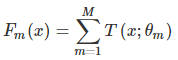模型一共训练M轮，每轮产生一个弱分类器 T(x;θm)T(x;θm)。弱分类器的损失函数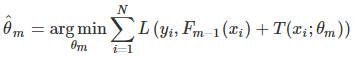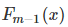为当前的模型，gbdt 通过经验风险极小化来确定下一个弱分类器的参数。具体到损失函数本身的选择也就是L的选择，有平方损失函数，0-1损失函数，对数损失函数等等。如果我们选择平方损失函数，那么这个差值其实就是我们平常所说的残差。

但是其实我们真正关注的，1.是希望损失函数能够不断的减小，2.是希望损失函数能够尽可能快的减小。所以如何尽可能快的减小呢？
让损失函数沿着梯度方向的下降。这个就是gbdt 的 gb的核心了。 利用损失函数的负梯度在当前模型的值作为回归问题提升树算法中的残差的近似值去拟合一个回归树。gbdt 每轮迭代的时候，都去拟合损失函数在当前模型下的负梯度。
这样每轮训练的时候都能够让损失函数尽可能快的减小，尽快的收敛达到局部最优解或者全局最优解。

gbdt 如何选择特征

GBDT中的弱分类器选择的是CART回归树。GBDT中特征的选择就是CART树的生成过程中特征属性的选择。而CART回归树的生成算法在这先不赘述，可参看李航的统计学习方法（后面有空再加篇笔记）。

gbdt 如何构建特征

其实说gbdt 能够构建特征并非很准确，gbdt 本身是不能产生特征的，但是我们可以利用gbdt去产生特征的组合。在CTR预估中，工业界一般会采用逻辑回归去进行处理, 逻辑回归本身是适合处理线性可分的数据，如果我们想让逻辑回归处理非线性的数据，其中一种方式便是组合不同特征，增强逻辑回归对非线性分布的拟合能力。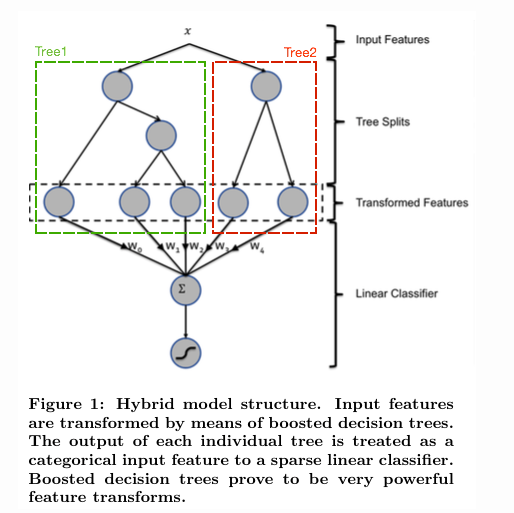图1：用GBDT 构造特征

如图 1所示，我们 使用 GBDT 生成了两棵树，两颗树一共有五个叶子节点。我们将样本 X 输入到两颗树当中去，样本X 落在了第一棵树的第二个叶子节点，第二颗树的第一个叶子节点，于是我们便可以依次构建一个五纬的特征向量，每一个纬度代表了一个叶子节点，样本落在这个叶子节点上面的话那么值为1，没有落在该叶子节点的话，那么值为 0.

于是对于该样本，我们可以得到一个向量[0,1,0,1,0] 作为该样本的组合特征，和原来的特征一起输入到逻辑回归当中进行训练。实验证明这样会得到比较显著的效果提升。

DT：回归树 Regression Decision Tree

GBDT并不是很多棵分类树。决策树分为两大类，回归树和分类树。前者用于预测实数值，如明天的温度、用户的年龄、网页的相关程度；后者用于分类标签值，如晴天/阴天/雾/雨、用户性别、网页是否是垃圾页面。这里要强调的是，前者的结果加减是有意义的，如10岁+5岁-3岁=12岁，后者则无意义，如男+男+女=到底是男是女？ GBDT的核心在于累加所有树的结果作为最终结果，就像前面对年龄的累加（-3是加负3），而分类树的结果显然是没办法累加的，所以GBDT中的树都是回归树，不是分类树，这点对理解GBDT相当重要（尽管GBDT调整后也可用于分类但不代表GBDT的树是分类树）。那么回归树是如何工作的呢？

下面我们以对人的性别判别/年龄预测为例来说明，每个instance都是一个我们已知性别/年龄的人，而feature则包括这个人上网的时长、上网的时段、网购所花的金额等。

作为对比，先说分类树，我们知道C4.5分类树在每次分枝时，是穷举每一个feature的每一个阈值，找到使得按照feature<=阈值，和feature>阈值分成的两个分枝的熵最大的feature和阈值（熵最大的概念可理解成尽可能每个分枝的男女比例都远离1:1），按照该标准分枝得到两个新节点，用同样方法继续分枝直到所有人都被分入性别唯一的叶子节点，或达到预设的终止条件，若最终叶子节点中的性别不唯一，则以多数人的性别作为该叶子节点的性别。

回归树总体流程也是类似，不过在每个节点（不一定是叶子节点）都会得一个预测值，以年龄为例，该预测值等于属于这个节点的所有人年龄的平均值。分枝时穷举每一个feature的每个阈值找最好的分割点，但衡量最好的标准不再是最大熵，而是最小化均方差--即（每个人的年龄-预测年龄）^2 的总和 / N，或者说是每个人的预测误差平方和 除以 N。这很好理解，被预测出错的人数越多，错的越离谱，均方差就越大，通过最小化均方差能够找到最靠谱的分枝依据。分枝直到每个叶子节点上人的年龄都唯一（这太难了）或者达到预设的终止条件（如叶子个数上限），若最终叶子节点上人的年龄不唯一，则以该节点上所有人的平均年龄做为该叶子节点的预测年龄。若还不明白可以Google "Regression Tree"，或阅读本文的第一篇论文中Regression Tree部分。

让损失函数沿着梯度方向的下降。这个就是gbdt 的 gb的核心。gbdt 每轮迭代的时候，都去拟合损失函数在当前模型下的负梯度。（如果损失函数使用的是平方误差损失函数，则这个损失函数的负梯度就可以用残差来代替，以下所说的残差拟合，便是使用了平方误差损失函数）

GBDT回归问题（预测）的例子

还是年龄预测，简单起见训练集只有4个人，A,B,C,D，他们的年龄分别是14,16,24,26。其中A、B分别是高一和高三学生；C,D分别是应届毕业生和工作两年的员工。如果是用一棵传统的回归决策树来训练，会得到如下图2所示结果：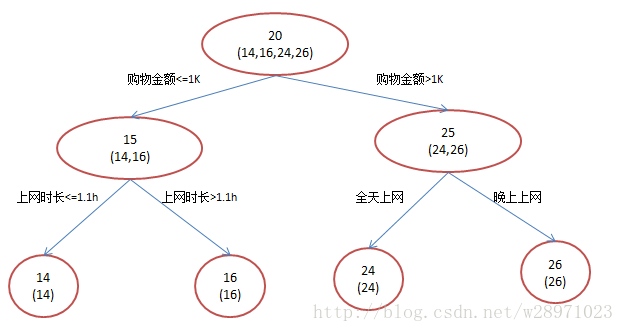图2：传统回归决策树

现在我们使用GBDT来做这件事，由于数据太少，我们限定叶子节点做多有两个，即每棵树都只有一个分枝，并且限定只学两棵树。我们会得到如下图3所示结果：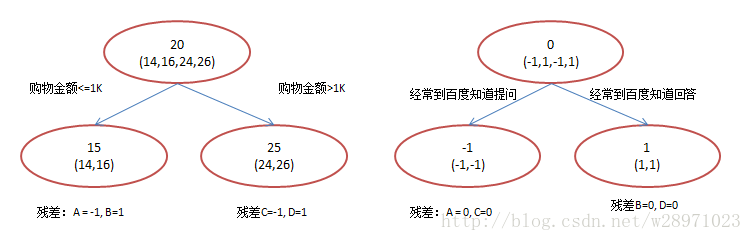图3：gbdt模型

在第一棵树分枝和图2一样，由于A,B年龄较为相近，C,D年龄较为相近，他们被分为两拨，每拨用平均年龄作为预测值。此时计算残差（残差的意思就是： A的预测值 + A的残差 = A的实际值），所以A的残差就是16-15=1（注意，A的预测值是指前面所有树累加的和，这里前面只有一棵树所以直接是15，如果还有树则需要都累加起来作为A的预测值）。进而得到A,B,C,D的残差分别为-1,1，-1,1。然后我们拿残差替代A,B,C,D的原值，到第二棵树去学习，如果我们的预测值和它们的残差相等，则只需把第二棵树的结论累加到第一棵树上就能得到真实年龄了。这里的数据显然是我可以做的，第二棵树只有两个值1和-1，直接分成两个节点。此时所有人的残差都是0，即每个人都得到了真实的预测值。

换句话说，现在A,B,C,D的预测值都和真实年龄一致了。Perfect!：

A: 14岁高一学生，购物较少，经常问学长问题；预测年龄A = 15 – 1 = 14

B: 16岁高三学生；购物较少，经常被学弟问问题；预测年龄B = 15 + 1 = 16

C: 24岁应届毕业生；购物较多，经常问师兄问题；预测年龄C = 25 – 1 = 24

D: 26岁工作两年员工；购物较多，经常被师弟问问题；预测年龄D = 25 + 1 = 26

讲到这里我们已经把GBDT最核心的概念、运算过程讲完了！没错就是这么简单。不过讲到这里很容易发现三个问题：

1）既然图2和图3 最终效果相同，为何还需要GBDT呢？

答案是过拟合。过拟合是指为了让训练集精度更高，学到了很多”仅在训练集上成立的规律“，导致换一个数据集当前规律就不适用了。其实只要允许一棵树的叶子节点足够多，训练集总是能训练到100%准确率的（大不了最后一个叶子上只有一个instance)。在训练精度和实际精度（或测试精度）之间，后者才是我们想要真正得到的。

我们发现图2为了达到100%精度使用了3个feature（上网时长、时段、网购金额），其中分枝“上网时长>1.1h” 很显然已经过拟合了，这个数据集上A,B也许恰好A每天上网1.09h, B上网1.05小时，但用上网时间是不是>1.1小时来判断所有人的年龄很显然是有悖常识的；

相对来说图3的boosting虽然用了两棵树 ，但其实只用了2个feature就搞定了，后一个feature是问答比例，显然图3的依据更靠谱。（当然，这里是LZ故意做的数据，所以才能靠谱得如此狗血。实际中靠谱不靠谱总是相对的） Boosting的最大好处在于，每一步的残差计算其实变相地增大了分错instance的权重，而已经分对的instance则都趋向于0。这样后面的树就能越来越专注那些前面被分错的instance。就像我们做互联网，总是先解决60%用户的需求凑合着，再解决35%用户的需求，最后才关注那5%人的需求，这样就能逐渐把产品做好，因为不同类型用户需求可能完全不同，需要分别独立分析。如果反过来做，或者刚上来就一定要做到尽善尽美，往往最终会竹篮打水一场空。

GBDT分类及分类问题例子

首先明确一点，gbdt 无论用于分类还是回归一直都是使用的CART 回归树。不会因为我们所选择的任务是分类任务就选用分类树，这里面的核心是因为gbdt 每轮的训练是在上一轮的训练的残差基础之上进行训练的。这里的残差就是当前模型的负梯度值 。这个要求每轮迭代的时候，弱分类器的输出的结果相减是有意义的。残差相减是有意义的。

如果选用的弱分类器是分类树，类别相减是没有意义的。上一轮输出的是样本 x 属于 A类，本一轮训练输出的是样本 x 属于 B类。 A 和 B 很多时候甚至都没有比较的意义，A 类- B类是没有意义的。

我们具体到分类这个任务上面来，我们假设样本 X 总共有 K类。来了一个样本 x，我们需要使用gbdt来判断 x 属于样本的哪一类。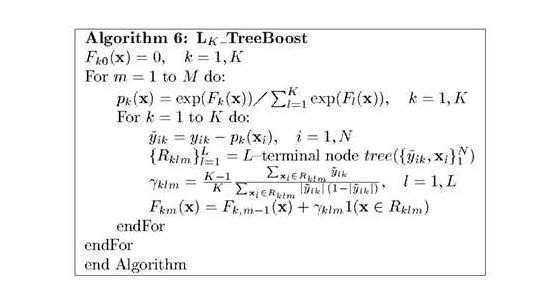图4：gbdt 多分类算法流程

第一步 我们在训练的时候，是针对样本 X 每个可能的类都训练一个分类回归树。举例说明，目前样本有三类，也就是 K = 3。样本 x 属于 第二类。那么针对该样本 x 的分类结果，其实我们可以用一个 三维向量 [0,1,0] 来表示。0表示样本不属于该类，1表示样本属于该类。由于样本已经属于第二类了，所以第二类对应的向量维度为1，其他位置为0。

针对样本有 三类的情况，我们实质上是在每轮的训练的时候是同时训练三颗树。第一颗树针对样本x的第一类，输入为（x,0）（x,0）。第二颗树输入针对 样本x 的第二类，输入为（x,1）（x,1）。第三颗树针对样本x 的第三类，输入为（x，0）（x，0）

在这里每颗树的训练过程其实就是就是我们之前已经提到过的CATR TREE 的生成过程。在此处我们参照之前的生成树的程序 即可以就解出三颗树，以及三颗树对x 类别的预测值f1(x),f2(x),f3(x)。那么在此类训练中，我们仿照多分类的逻辑回归 ，使用softmax 来产生概率，则属于类别 1 的概率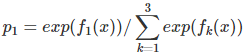并且我们我们可以针对类别1 求出 残差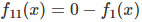; 类别2 求出残差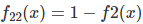；类别3 求出残差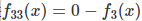。 然后开始第二轮训练 针对第一类 输入为（x,f11(x)）, 针对第二类输入为（x,f22(x)), 针对 第三类输入为 (x,f33(x)).继续训练出三颗树。一直迭代M轮。每轮构建 3颗树。

所以当K =3。我们其实应该有三个式子：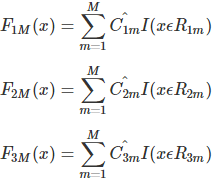当训练完毕以后，新来一个样本 x1 ，我们需要预测该样本的类别的时候，便可以有这三个式子产生三个值，f1(x),f2(x),f3(x)。样本属于 某个类别c的概率为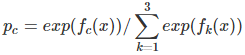上面的理论阐述可能仍旧过于难懂，我们下面将拿Iris 数据集中的六个数据作为例子，来展示gbdt 多分类的过程。

样本编号
花萼长度(cm)
花萼宽度(cm)
花瓣长度(cm)
花瓣宽度
花的种类
1
5.1
3.5
1.4
0.2
山鸢尾
2
4.9
3.0
1.4
0.2
山鸢尾
3
7.0
3.2
4.7
1.4
杂色鸢尾
4
6.4
3.2
4.5
1.5
杂色鸢尾
5
6.3
3.3
6.0
2.5
维吉尼亚鸢尾
6
5.8
2.7
5.1
1.9
维吉尼亚鸢尾
表1： Iris 数据集

这是一个有6个样本的三分类问题。我们需要根据这个花的花萼长度，花萼宽度，花瓣长度，花瓣宽度来判断这个花属于山鸢尾，杂色鸢尾，还是维吉尼亚鸢尾。具体应用到gbdt多分类算法上面。我们用一个三维向量来标志样本的label。[1,0,0] 表示样本属于山鸢尾，[0,1,0] 表示样本属于杂色鸢尾，[0,0,1] 表示属于维吉尼亚鸢尾。

gbdt 的多分类是针对每个类都独立训练一个 CART Tree。所以这里，我们将针对山鸢尾类别训练一个 CART Tree 1。杂色鸢尾训练一个 CART Tree 2 。维吉尼亚鸢尾训练一个CART Tree 3，这三个树相互独立。

我们以样本 1 为例。针对 CART Tree1 的训练样本是[5.1,3.5,1.4,0.2]，label 是 1，最终输入到模型当中的为[5.1,3.5,1.4,0.2,1]。针对 CART Tree2 的训练样本也是[5.1,3.5,1.4,0.2],但是label 为 0,最终输入模型的为[5.1,3.5,1.4,0.2,0]. 针对 CART Tree 3的训练样本也是[5.1,3.5,1.4,0.2],label 也为0,最终输入模型当中的为[5.1,3.5,1.4,0.2,0].

下面我们来看 CART Tree1 是如何生成的，其他树 CART Tree2 , CART Tree 3的生成方式是一样的。CART Tree的生成过程是从这四个特征中找一个特征做为CART Tree1 的节点。比如花萼长度做为节点。6个样本当中花萼长度 大于5.1 cm的就是 A类，小于等于 5.1 cm 的是B类。生成的过程其实非常简单，问题 1.是哪个特征最合适？ 2.是这个特征的什么特征值作为切分点？ 即使我们已经确定了花萼长度做为节点。花萼长度本身也有很多值。在这里我们的方式是遍历所有的可能性，找到一个最好的特征和它对应的最优特征值可以让当前式子的值最小。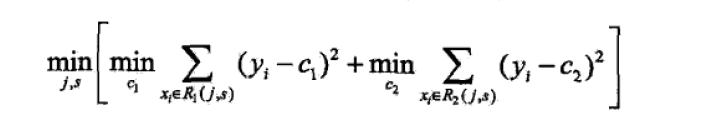我们以第一个特征的第一个特征值为例。R1 为所有样本中花萼长度小于 5.1 cm 的样本集合，R2 为所有样本当中花萼长度大于等于 5.1cm 的样本集合。所以 R1={2}R1={2}，R2={1,3,4,5,6}R2={1,3,4,5,6}.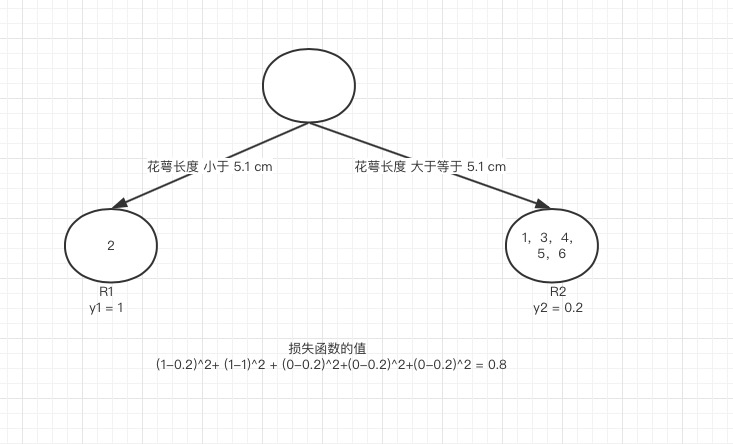图 5 节点分裂示意图

y1 为 R1 所有样本的label 的均值 1/1=11/1=1。y2 为 R2 所有样本的label 的均值 (1+0+0+0+0)/5=0.2(1+0+0+0+0)/5=0.2。

下面便开始针对所有的样本计算这个式子的值。样本1 属于 R2 计算的值为(1−0.2)2(1−0.2)2, 样本2 属于R1 计算的值为(1−1)2(1−1)2, 样本 3，4，5，6同理都是 属于 R2的 所以值是(0−0.2)2(0−0.2)2. 把这六个值加起来，便是 山鸢尾类型在特征1 的第一个特征值的损失值。这里算出来(1-0.2)^2+ (1-1)^2 + (0-0.2)^2+(0-0.2)^2+(0-0.2)^2 = 0.8.

接着我们计算第一个特征的第二个特征值，计算方式同上，R1 为所有样本中 花萼长度小于 4.9 cm 的样本集合，R2 为所有样本当中 花萼长度大于等于 4.9 cm 的样本集合.所以 R1={}R1={}，R1={1,2,3,4,5,6}R1={1,2,3,4,5,6}. y1 为 R1 所有样本的label 的均值 = 0。y2 为 R2 所有样本的label 的均值 (1+1+0+0+0+0)/6=0.3333(1+1+0+0+0+0)/6=0.3333。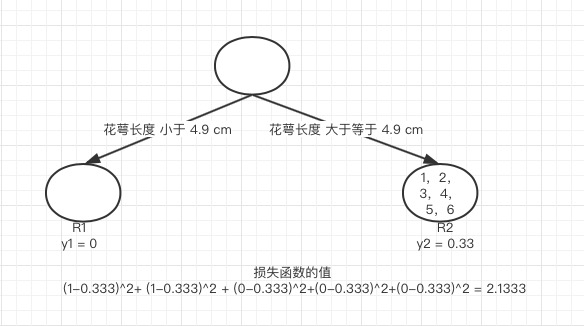图 6 第一个特征的第二个特侦值的节点分裂情况

我们需要针对所有的样本,样本1 属于 R2, 计算的值为(1−0.333)^2, 样本2 属于R2 ,计算的值为(1−0.333)^2, 样本 3，4，5，6同理都是 属于 R2的, 所以值是(0−0.333)^2. 把这六个值加起来山鸢尾类型在特征1 的第二个特征值的损失值。这里算出来 (1-0.333)^2+ (1-0.333)^2 + (0-0.333)^2+(0-0.333)^2+(0-0.333)^2 = 2.1333. 这里的损失值大于 特征一的第一个特征值的损失值，所以我们不取这个特征的特征值。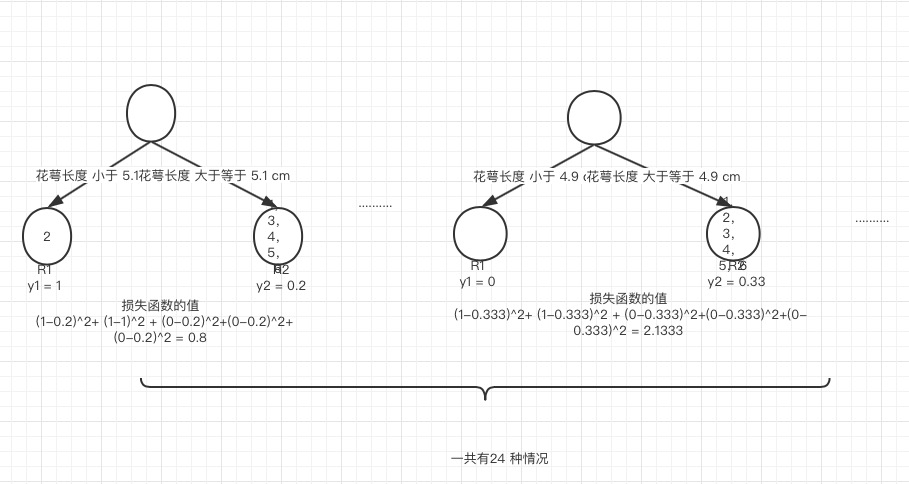图 7 所有情况说明

这样我们可以遍历所有特征的所有特征值，找到让这个式子最小的特征以及其对应的特征值，一共有24种情况,4个特征*每个特征有6个特征值。在这里我们算出来让这个式子最小的特征花萼长度,特征值为5.1 cm。这个时候损失函数最小为 0.8。

于是我们的预测函数此时也可以得到: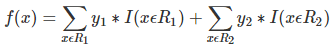此处 R1 = {2},R2 = {1,3,4,5,6}，y1 = 1,y2 = 0.2。训练完以后的最终式子为: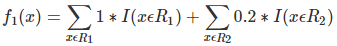借由这个式子，我们得到对样本属于类别1 的预测值 f1(x)=1+0.2∗5=2。同理我们可以得到对样本属于类别2,3的预测值f2(x),f3(x).样本属于类别1的概率 即为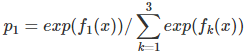展开全文• ## 集成树之三：GBDT

万次阅读 2019-08-21 14:36:25机器学习 GBDT
• ## GBDT

千次阅读 2019-06-17 16:32:39
什么是GBDTGBDT(梯度提升树)，是一个以回归树为基学习器，以boost为框架的加法模型的集成学习。 GBDT基于GB算法。GB算法的主要思想是，每次建立模型是在之前建立模型损失函数的梯度下降方向。损失函数是评价...
• 1、GBDT 2、GBDT思想 3、负梯度拟合 4、损失函数 4.1、分类 4.2、回归 5、GBDT回归算法 6、GBDT分类算法 6.1、二分类 6.2、多分类 7、正则化 8、RF与GBDT之间的区别与联系 9、优缺点 优点 缺点 10、...
• ## GBDT问题汇总

千次阅读 2018-08-30 09:41:09
GBDT几问 本篇文章主要介绍GBDT基本原理以及一些细节性的东西，这些东西更多在面试使用，或者对于二次创新使用，主要内容有以下几个方面：   GBDT几问 Boosting算法Bagging算法介绍 GBDT基本原理 ...
• ## GBDT算法原理

千次阅读 2020-07-14 23:49:06
一、基础知识 1.泰勒级数展开 2.梯度下降法 3.牛顿法 4.从参数空间到函数空间 二、GBDT 1 .DT：回归树 Regression Decision Tree 5.GBDT 适用范围 2. GB：梯度迭代 Gradient Boosting ...
• ## gbdt+fm

千次阅读 2016-05-07 23:10:44
https://github.com/guestwalk/kaggle-2014-criteo
• ## GBDT算法的优缺点

千次阅读 2017-05-21 17:18:20
优点： 预测精度高 适合低维数据 能处理非线性数据 缺点： 并行麻烦（因为上下两棵树有联系） 如果数据维度较高时会加大算法的计算复杂度
• 知乎回答：LR,gbdt,libfm这三种模型分别适合处理什么类型的特征,为了取得较好效果他们对特征有何要求？ https://www.zhihu.com/question/35821566 参考博客：这些经典模型的优缺点 ...
• （1）如何在numpy数组中选取若干列或者行？ >>>import numpy as np >>>tmp_a = np.array([[1,1], [0.4, 4], [1., 0.9]])  >>>tmp_a >>>tmp_a[[0,1],:]#选第0、1行  ...>>>tmp_a[np.array([True, False, True]),:...sklearn 机器学习 Python GBDT 特征选择
• ## GBDT和随机森林的区别

万次阅读 多人点赞 2017-01-22 11:55:00
GBDT和随机森林的相同点： 1、都是由多棵树组成 2、最终的结果都是由多棵树一起决定 GBDT和随机森林的不同点： 1、组成随机森林的树可以是分类树，也可以是回归树；而GBDT只由回归树组成 2、组成随机森林的树可以...机器学习 决策树 GBDT 数据挖掘 算法
• ## GBDT构建组合特征

千次阅读 2017-05-18 14:19:48
动机在于GBDT无法直接处理海量的离散特征，复杂度太高，所以主要思路就是就是先用已有特征训练GBDT模型，然后利用GBDT模型学习到的树来构造新特征，最后把这些新特征加入原有特征一起训练模型，事实上就是一种...
• ## GBDT常用损失函数

千次阅读 2019-11-03 18:03:26
分类算法的损失函数： 指数损失函数 对数损失函数： 二元分类的对数函数 多元分类的对数函数 回归算法的损失函数： 均方损失函数 ...还需要好好整理一下，常用算法的 损失函数做到随时都能写出来 ...
• ## GBDT(sklearn)进行回归

千次阅读 2018-06-04 13:43:04
sklearn中可以使用GBDT进行分类和回归，下面是GBDT进行回归的文档...gbdt
• GBDT 及其改进版本（XGboost, lightGBM）在数据竞赛中占了大半江山，网上讲解的文章也很多，但大多是文字和公式的形式，这里尝试用简单的图解形式，去理解 GBDT 的基本操作过程。 参考《统计学习方法》P149中的例子...GBDT
• ## GBDT浅谈以及代码实现

万次阅读 多人点赞 2017-04-21 16:03:54
GBDT作为近年很热门的模型，其性能非常突出，用途也是涵盖了从特征选择到分类、回归，被广大从业者和爱好者所使用。 网上关于gbdt的原理和数学推导已经有很多，我就谈谈我个人的浅见，如有错误还望指正。同时还附上...python 算法 gbdt
...